## 1. 题目描述（中等难度）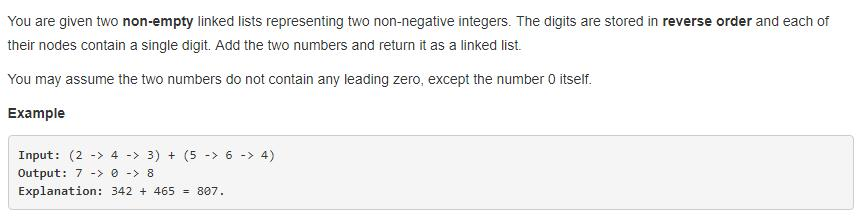## 2. 图示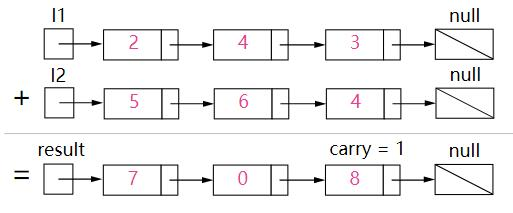## 3. 思路

• 初始化一个节点的头，dummy head ，但是这个头不存储数字。并且将 curr 指向它。
• 初始化进位 carry 为 0 。
• 初始化 p 和 q 分别为给定的两个链表 l1 和 l2 的头，也就是个位。
• 循环，直到 l1 和 l2 全部到达 null 。
• 设置 x 为 p 节点的值，如果 p 已经到达了 null，设置 x 为 0 。
• 设置 y 为 q 节点的值，如果 q 已经到达了 null，设置 y 为 0 。
• 设置 sum = x + y + carry 。
• 更新 carry = sum / 10 。
• 创建一个值为 sum mod 10 的节点，并将 curr 的 next 指向它，同时 curr 指向变为当前的新节点。
• 向前移动 p 和 q 。
• 判断 carry 是否等于 1 ，如果等于 1 ，在链表末尾增加一个为 1 的节点。
• 返回 dummy head 的 next ，也就是个位数开始的地方。

## 4. 代码

class ListNode {
int val;
ListNode next;
ListNode(int x) { val = x; }
}
public ListNode addTwoNumbers(ListNode l1, ListNode l2) {
ListNode p = l1, q = l2, curr = dummyHead;
int carry = 0;
while (p != null || q != null) {
int x = (p != null) ? p.val : 0;
int y = (q != null) ? q.val : 0;
int sum = carry + x + y;
carry = sum / 10;
curr.next = new ListNode(sum % 10);
curr = curr.next;
if (p != null) p = p.next;
if (q != null) q = q.next;
}
if (carry > 0) {
curr.next = new ListNode(carry);
}
}


## 5. 扩展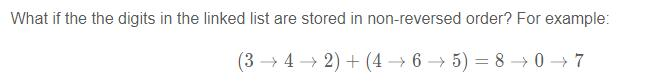## 6. 迭代思想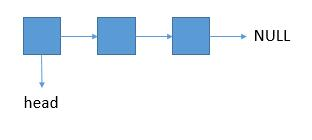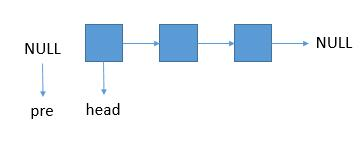• next 指向 head 的 next ，防止原链表丢失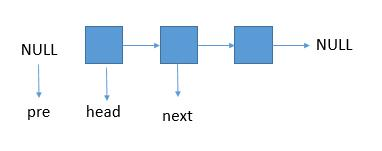• head 的 next 从原来链表脱离，指向 pre 。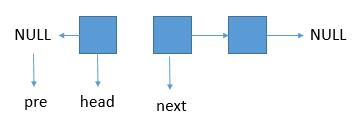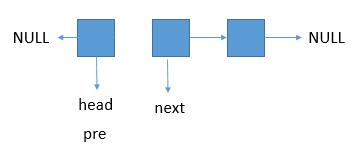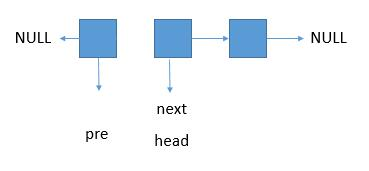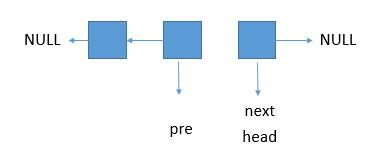next = head -> next; //保存 head 的 next , 以防取下 head 后丢失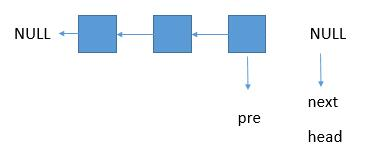## 7. 迭代代码

public ListNode reverseList(ListNode head){
ListNode pre=null;
ListNode next;
}
return pre;
}


## 8. 递归思想

• 接着我们确定如何把问题一步一步的化小，我们可以这样想。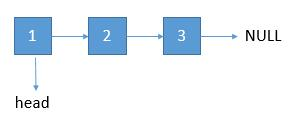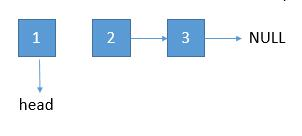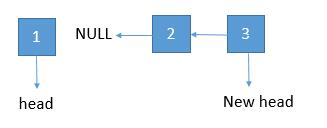• 将 2 指向 1 ，1 指向 null，将 newhead 返回即可。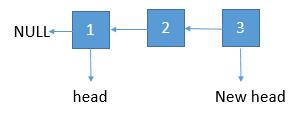• 找到递归出口

当然就是如果结点的个数是一个，那么逆序的话还是它本身，直接 return 就够了。怎么判断结点个数是不是一个呢？它的 next 等于 null 就说明是一个了。但如果传进来的本身就是 null，那么直接找它的 next 会报错，所以先判断传进来的是不是 null ，如果是，也是直接返回就可以了。

## 9. 代码

public ListNode reverseListRecursion(ListNode head){